# Angle Sum Theorem

Angle Sum Theorem

Austin is a civil engineer.

He is trying to figure out the measurements of all angles of a roof which is in the form of a triangle.He knows one angle is of $$45^{\circ}$$ and the other is a right angle.

Can you help him to figure out the measurement of the third angle?

To answer this, you need to understand the angle sum theorem, which is a remarkable property of a triangle and connects all its three angles.

In this mini-lesson, we will explore the world of the angle sum theorem.

You will get to learn about the triangle angle sum theorem definition, exterior angle sum theorem, polygon exterior angle sum theorem, polygon angle sum theorem, and discover other interesting aspects of it.

You can check out the interactive simulations to know more about the lesson and try your hand at solving a few interesting practice questions at the end of the page.

## Lesson Plan

 1 What Is the Definition of Angle Sum Theorem? 2 Important Notes on Angle Sum Theorem 3 Solved Examples on Angle Sum Theorem 4 Thinking out of the Box! 5 Interactive Questions on Angle Sum Theorem

## What Is the Definition of Angle Sum Theorem?

### Angle Sum Theorem Definition

In any triangle, the sum of the three angles is $$180^{\circ}$$.

In $$\Delta ABC$$, $$\angle A + \angle B+ \angle C=180^{\circ}$$.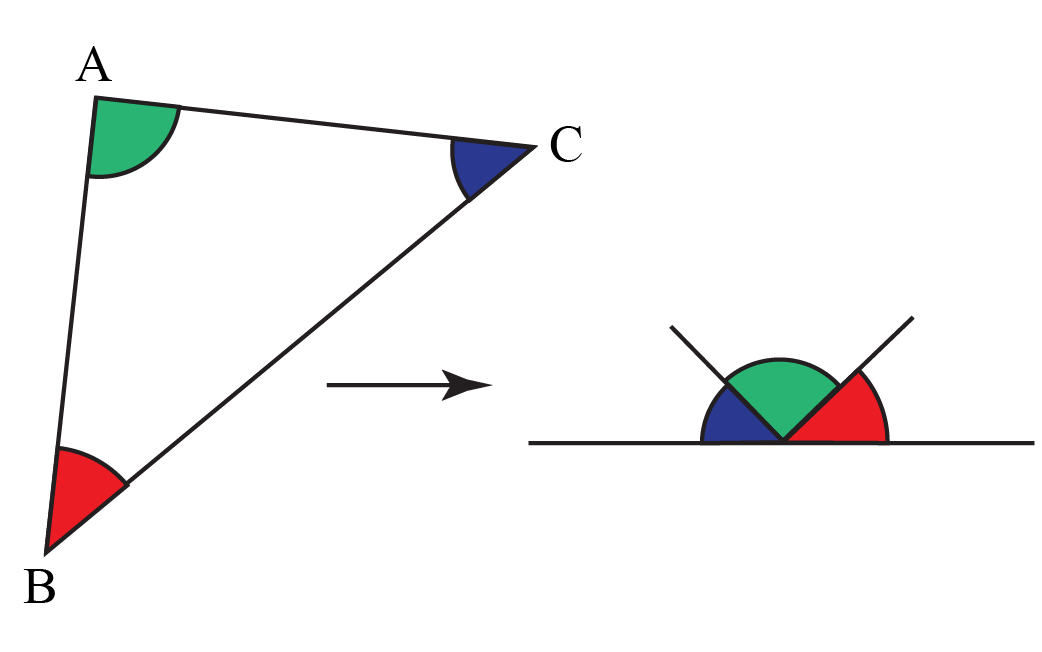### Angle Sum Theorem Formula

So, the angle sum theorem formula can be given as:

 $\angle A + \angle B+ \angle C=180^{\circ}$

## What Is the Angle Sum Theorem?

Let us perform two activities to understand the angle sum theorem.

### Activity 1

1. Draw any triangle on a piece of paper.

2. Cut all three angles of the triangle.

3. Rearrange these angles as shown below.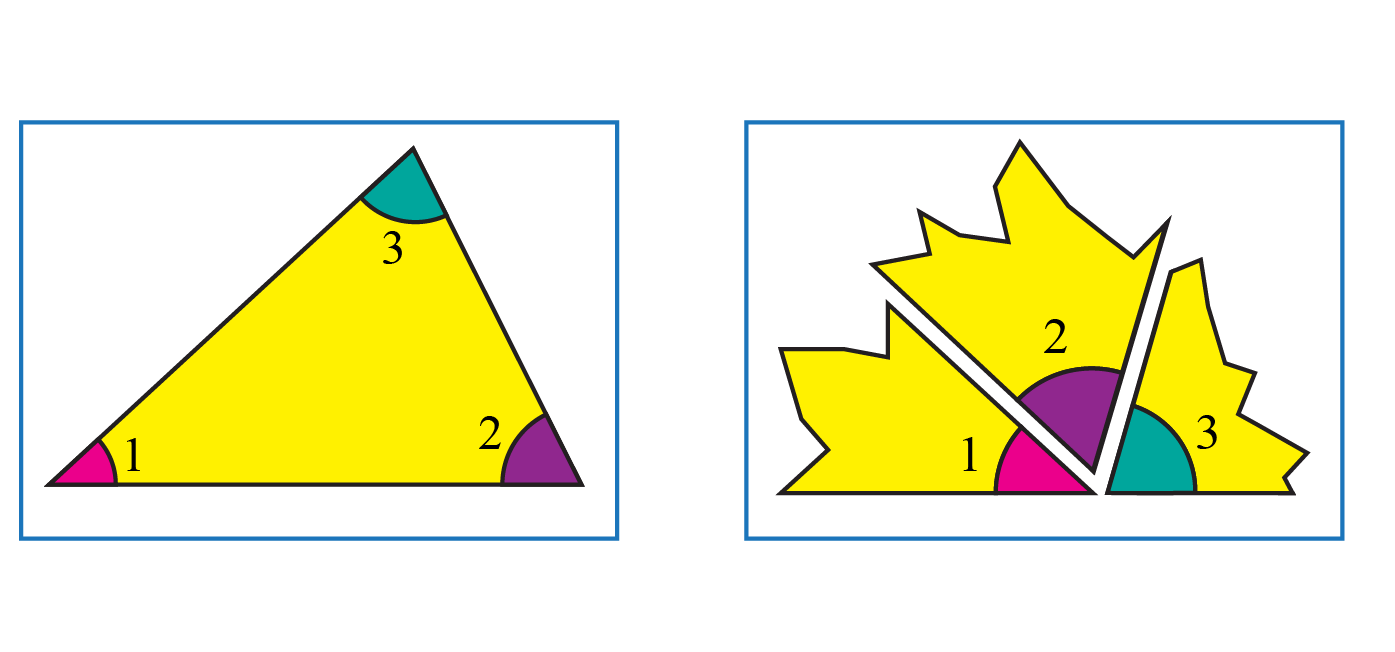Did you notice that all three angles constitute one straight angle?

So, $$\angle 1 + \angle 2+ \angle 3=180^{\circ}$$.

You can visualize this activity using the simulation below.

### Activity 2

1. Draw three copies of one triangle on a piece of paper.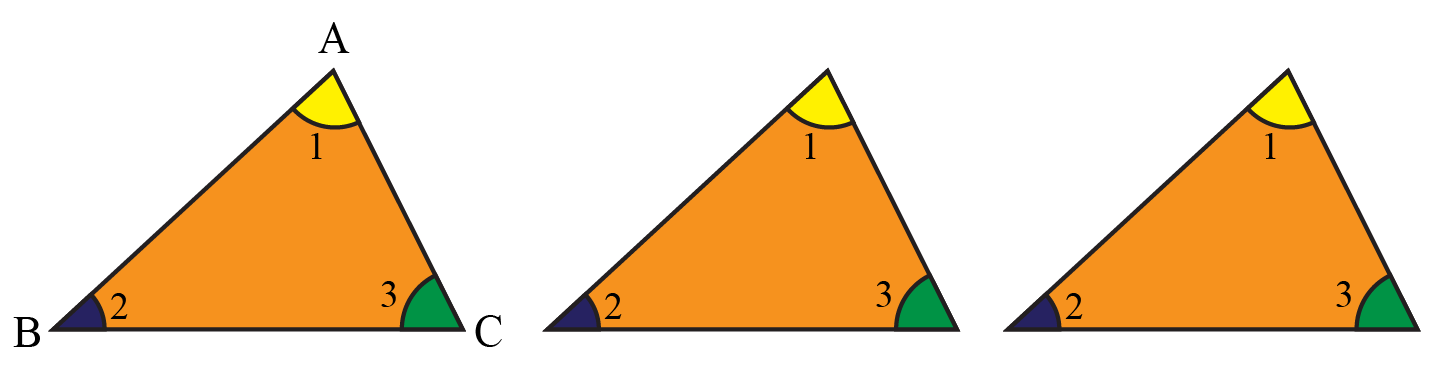2. Arrange these triangles as shown below.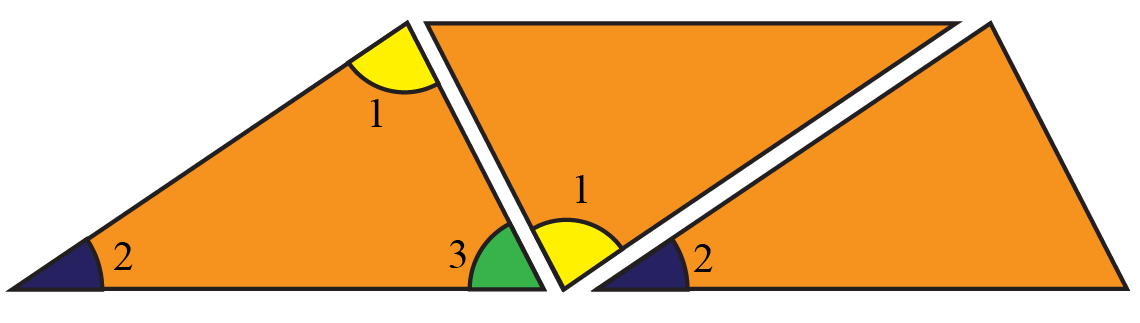Again observe that these three angles constitute a straight angle.

You can visualize this activity using the simulation below.

Drag the slider to observe each step.

### Proof

Let $$\angle 1, \angle 2$$, and $$\angle 3$$ be the angles of $$\Delta ABC$$.

Let $$\angle 4$$ be the exterior angle.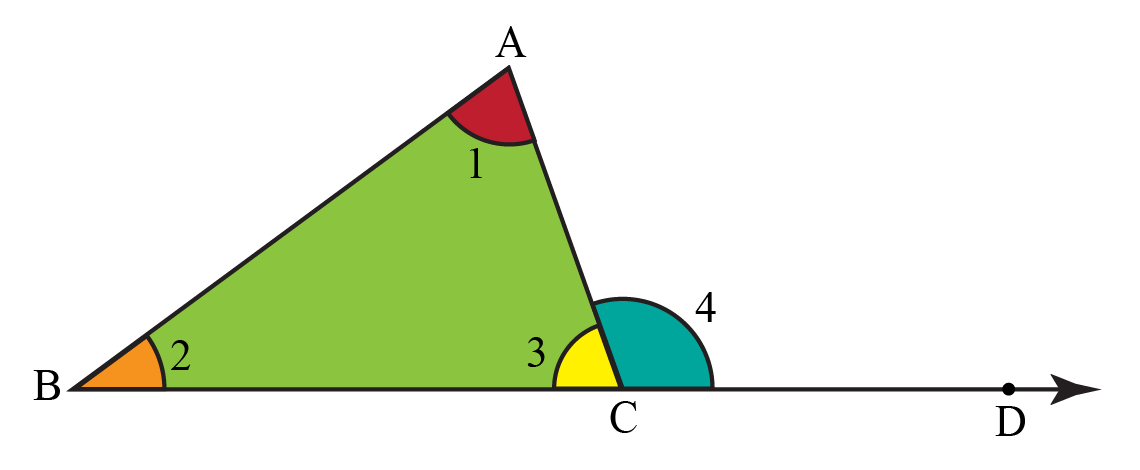Then, by exterior angle sum theorem, we have $$\angle 1+\angle 2=\angle 4$$.

Adding $$\angle 3$$ on both sides of this equation, we get $$\angle 1+\angle 2+\angle 3=\angle 4+\angle 3$$.

$$\angle 4$$ and $$\angle 3$$ form a pair of supplementary angles because it is a linear pair.

So, $$\angle 1+\angle 2+\angle 3=180^{\circ}$$.

## What Is the Exterior Angle Sum Theorem?

Exterior angle sum theorem states that "an exterior angle of a triangle is equal to the sum of its two interior opposite angles."

Let's do an activity to prove it.

Take a piece of paper and draw a triangle ABC on it.

Extend one of the sides, say, BC.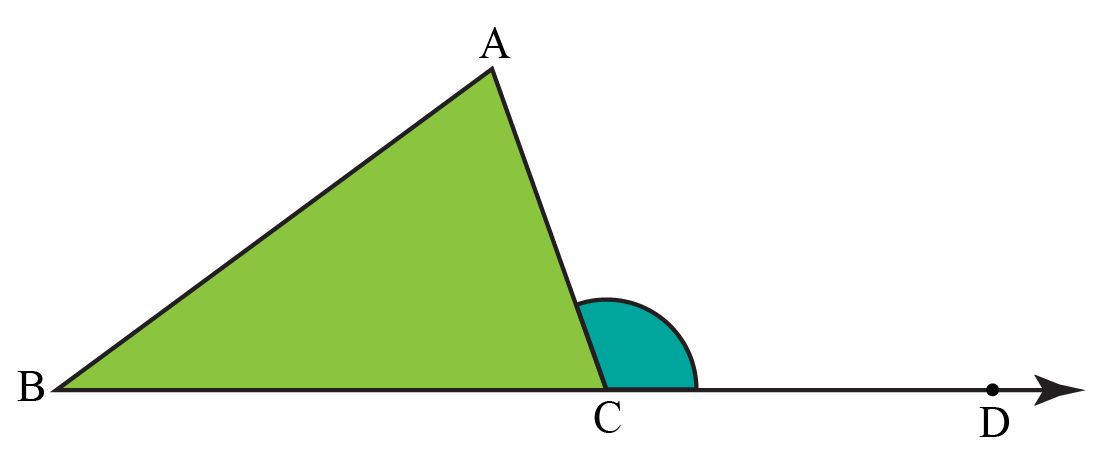Here, $$\angle ACD$$ is an exterior angle of $$\Delta ABC$$.

$$\angle A$$ and $$\angle B$$ are the two opposite interior angles of $$\angle ACD$$.

Cut out these two angles and place them together as shown below.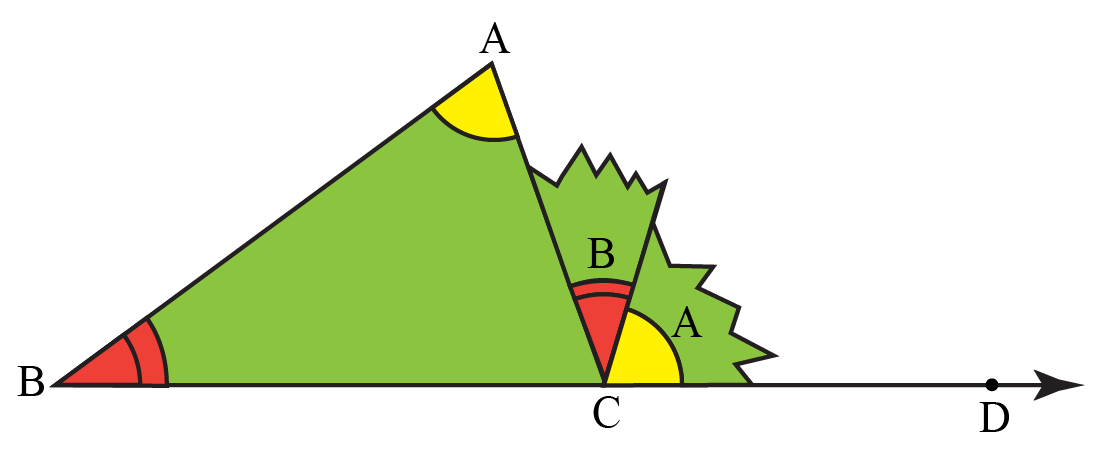Do these two angles cover $$\angle ACD$$ completely?

So, we can say that $$\angle ACD=\angle A+\angle B$$.

You can visualize this activity using the simulation below.

## What Is Polygon Exterior Angle Sum Theorem?

The polygon exterior angle sum theorem states that "the sum of all exterior angles of a convex polygon is equal to $$360^{\circ}$$."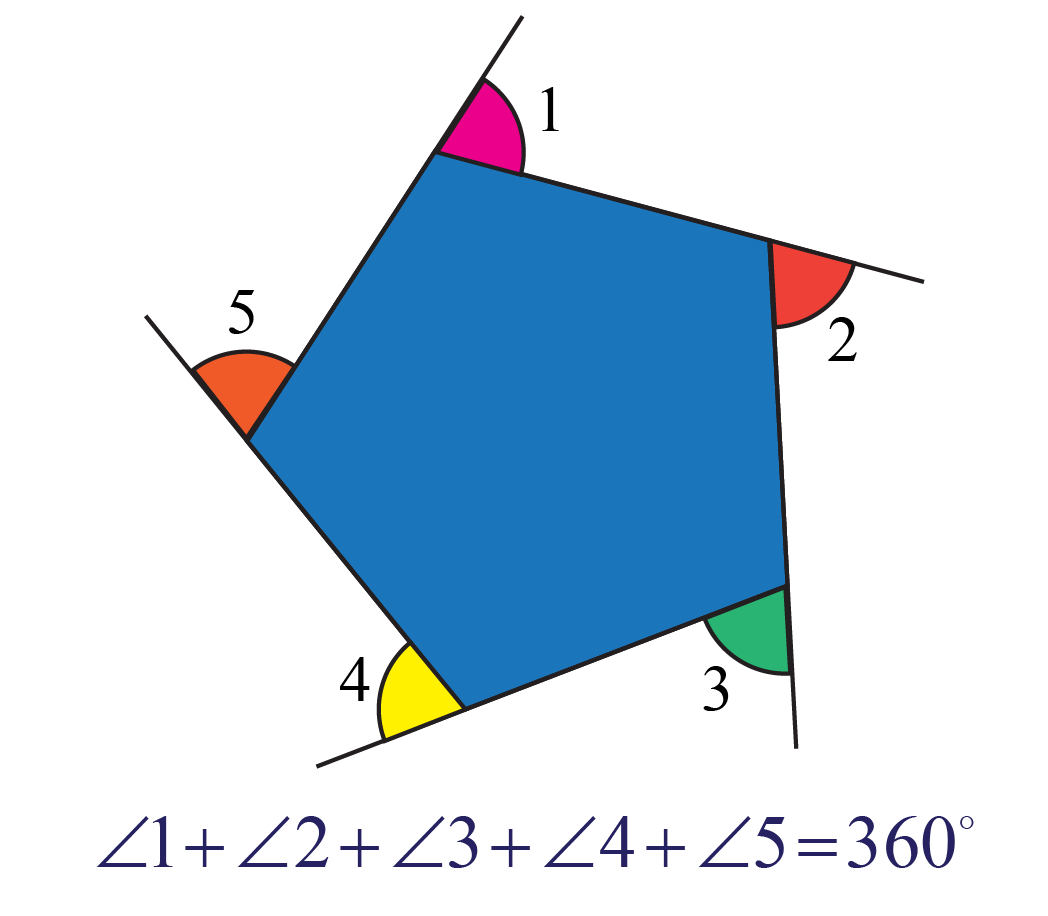Observe that in this 5-sided polygon, the sum of all exterior angles is $$360^{\circ}$$ by polygon angle sum theorem.

## Solved Examples

 Example 1

Ms Amy asked her students which of the following can be the angles of a triangle?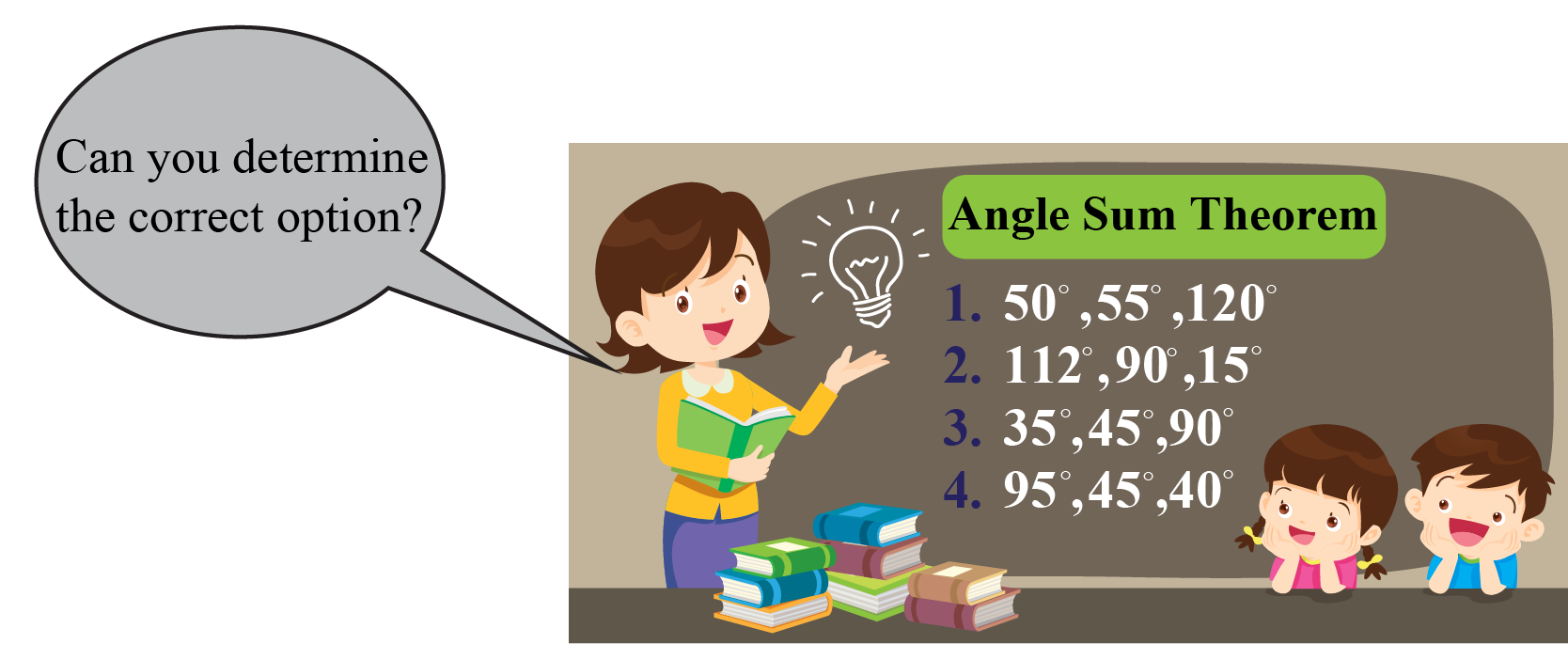Can you determine the angles?

Solution

We will check each option by finding the sum of all three angles.

In the first option, we have angles $$50^{\circ},55^{\circ}$$, and $$120^{\circ}$$.

The sum is $$50^{\circ}+55^{\circ}+120^{\circ}=225^{\circ}>180^{\circ}$$.

In the second option, we have angles $$112^{\circ}, 90^{\circ}$$, and $$15^{\circ}$$.

The sum is $$112^{\circ}+90^{\circ}+15^{\circ}=217^{\circ}>180^{\circ}$$.

In the third option, we have angles $$35^{\circ}, 45^{\circ}$$, and $$40^{\circ}$$.

The sum is $$35^{\circ}+45^{\circ}+90^{\circ}=170^{\circ}<180^{\circ}$$.

In the fourth option, we have angles $$95^{\circ}, 45^{\circ}$$, and $$40^{\circ}$$.

The sum is $$95^{\circ}+45^{\circ}+40^{\circ}=180^{\circ}$$.

So, only the fourth option gives the sum of $$180^{\circ}$$.

 $$\therefore$$ The fourth option is correct.
 Example 2

Look at the figure shown below.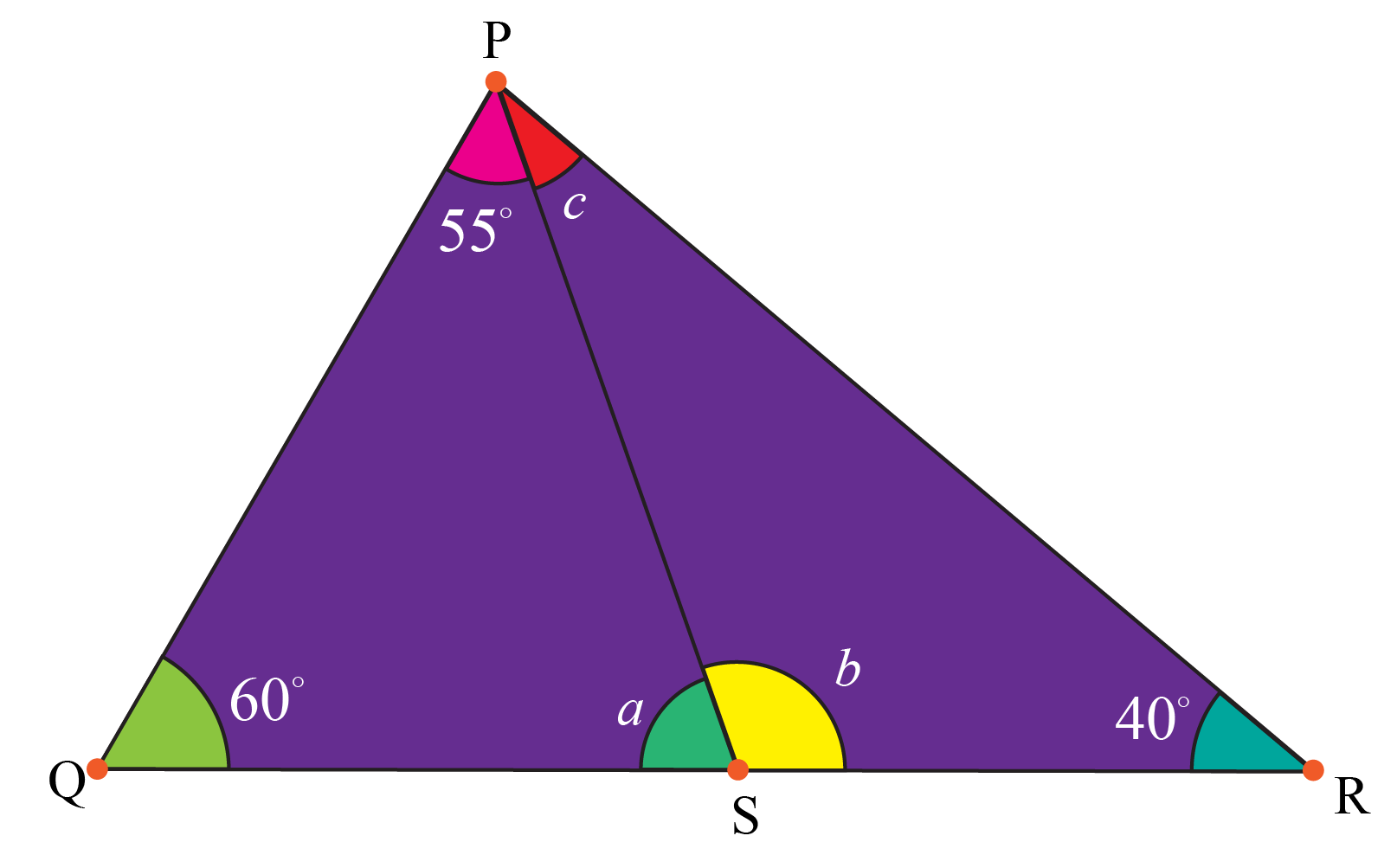Can you find the missing angles $$a$$, $$b$$, and $$c$$?

Solution

In $$\Delta PQS$$, we will apply the triangle angle sum theorem to find the value of $$a$$.

\begin{align}\angle PQS+\angle QPS+\angle PSQ&=180^{\circ}\\60^{\circ}+55^{\circ}+a&=180^{\circ}\\115^{\circ}+a&=180^{\circ}\\a&=65^{\circ}\end{align}

We can find the value of $$b$$ by using the definition of a linear pair.

\begin{align}\angle PSR+\angle PSQ&=180^{\circ}\\b+65^{\circ}&=180^{\circ}\\b&=115^{\circ}\end{align}

In $$\Delta PQS$$, we will apply the triangle angle sum theorem to find the value of $$c$$.

\begin{align}\angle PSR+\angle PRS+\angle SPR&=180^{\circ}\\115^{\circ}+40^{\circ}+c&=180^{\circ}\\155^{\circ}+c&=180^{\circ}\\c&=25^{\circ}\end{align}

 $$a=65^{\circ}, b=115^{\circ}$$ and $$c=25^{\circ}$$
 Example 3

One of the acute angles of a right-angled triangle is $$45^{\circ}$$.

Find the other angle.

Identify the type of triangle thus formed.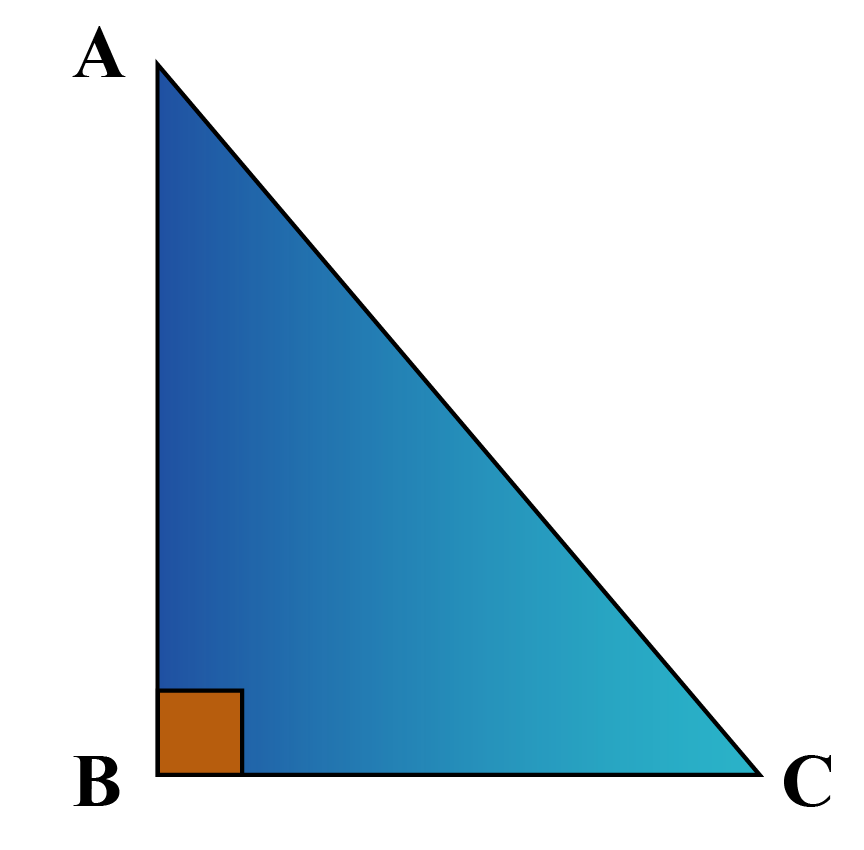Solution

Given, angle1 =  90° and angle2 = 45°

We know that the sum of the angles of a triangle adds up to 180°.

Therefore,

\begin{align} \text{angle}_3 &=180^{\circ}-(90^{\circ} +45^{\circ}) \\ &= 45^{\circ}\end{align}

Since two angles measure the same, it is an isosceles triangle.

 $$\therefore$$ $$\Delta ABC$$ is an isosceles triangle.Think Tank
 1. Frank has two secret lucky numbers, $$x$$ and $$y$$, with him. He gives a few hints in the form of a figure to his friend Joe to crack it.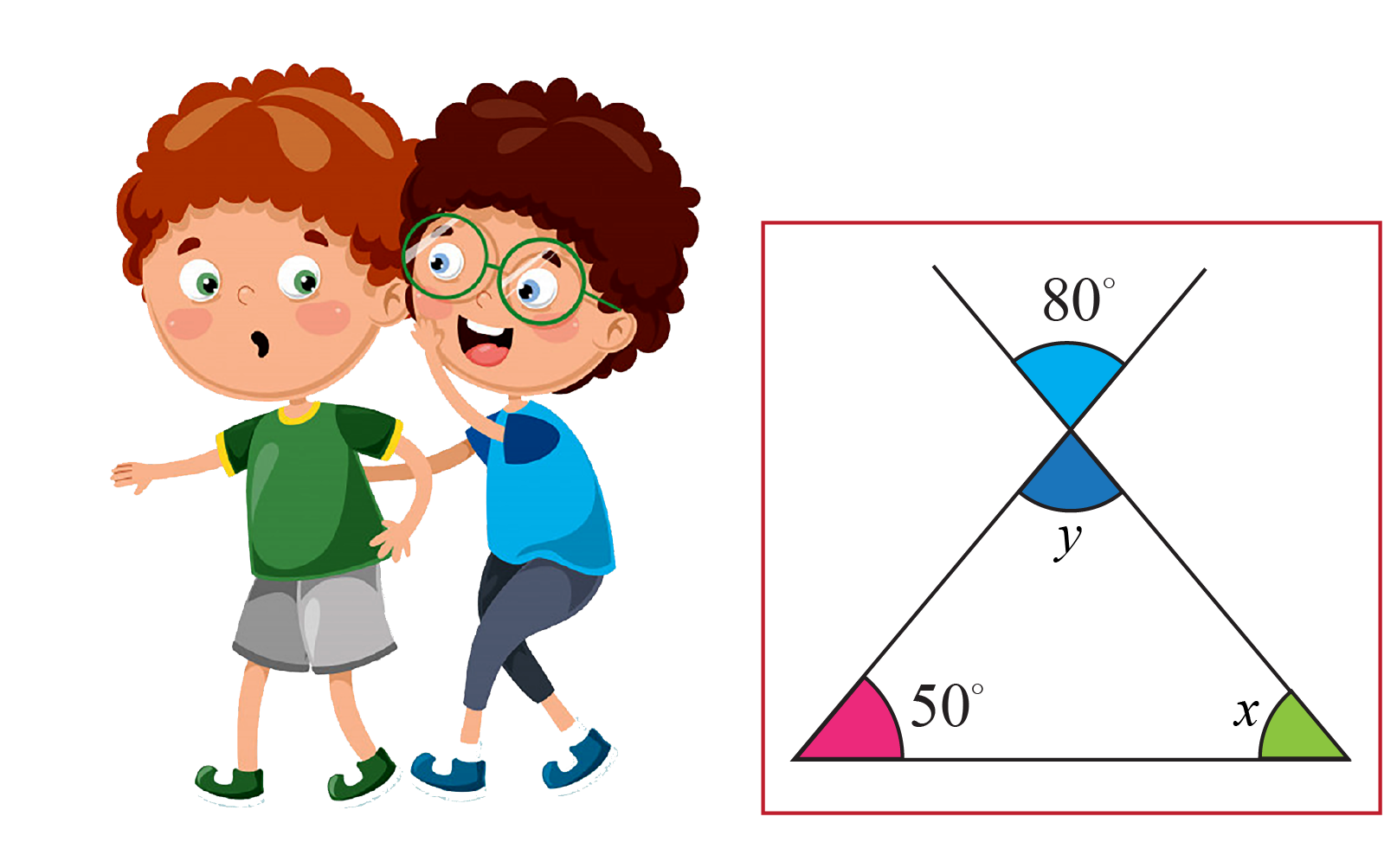Can you determine these two lucky numbers?

## Interactive Questions

Here are a few activities for you to practice. Select/type your answer and click the "Check Answer" button to see the result.

## Let's Summarize

This mini-lesson targeted the fascinating concept of the Angle Sum Theorem. The math journey around Angle Sum Theorem starts with what a student already knows, and goes on to creatively crafting a fresh concept in the young minds. Done in a way that is not only relatable and easy to grasp, but will also stay with them forever. Here lies the magic with Cuemath.

## About Cuemath

At Cuemath, our team of math experts are dedicated to making learning fun for our favorite readers, the students! Through an interactive and engaging learning-teaching-learning approach, the teachers explore all angles of a topic. Be it problems, online classes, doubt sessions, or any other form of relation, it’s the logical thinking and smart learning approach that we, at Cuemath, believe in.

## FAQs on Angle Sum Theorem

### 1. How do you find the angle sum theorem?

The angle sum theorem can be found using the statement "The sum of all interior angles of a triangle is equal to $$180^{\circ}$$."

### 2. What is the angle sum theorem for quadrilaterals?

The angle sum theorem for quadrilaterals is that the sum of all measures of angles of the quadrilateral is $$360^{\circ}$$.

### 3. What is the angle sum property?

The angle sum property of a triangle states that the sum of the three angles is $$180^{\circ}$$.

### 4. Why the sum of angles of a triangle is 180?

The sum of all angles of a triangle is $$180^{\circ}$$ because one exterior angle of the triangle is equal to the sum of opposite interior angles of the triangle.

### 5. What is the polygon angle sum theorem?

The polygon exterior angle sum theorem states that "the sum of all exterior angles of a convex polygon is equal to $$360^{\circ}$$."

### 6. What is the sum of 3 angles of a triangle?

The sum of 3 angles of a triangle is $$180^{\circ}$$.

### 7. What is the sum of all angles of a triangle?

The sum of all angles of a triangle is $$180^{\circ}$$.

More Important Topics
Numbers
Algebra
Geometry
Measurement
Money
Data
Trigonometry
Calculus